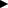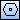# Creating Regular Polygon

The following 3 methods can be used to create a regular polygon.

• Creating an inscribed/circumscribed polygon
• Creating a polygon by specifying the length per side
• Creating a polygon by specifying two vertexes

To display a command parameter to be executed, click/of command parameters, or press Tab key or / key on the keyboard.

1. Point [Polygon] on [Shape] menu, and click [Regular Polygon].
Then you will be able to create a regular polygon.
2.  Supplemental ▪ You can enter into the regular polygon creating status by clickingon [Shape] toolbar.
3. Input the number of sides for regular polygons to be created in [Side Count] box on Command Window.
4. Specify a method to create a regular polygon in [Specifying Method] box on Command Window.
Inscribed
Creates a regular polygon Inscribed to a circle with the specified radius.
Circumscribed
Creates a regular polygon circumscribed to a circle with the specified radius.
Side Length
Creates a regular polygon with the specified side length.
Vertex
Creates a regular polygon using a line connecting the specified 2 vertexes as the length per side.

### When creating an inscribed/circumscribed polygon

1. Click the center point of a circle on the drawing.
2. Specify the radius of the circle.
When specifying a point to decide radius
Switch the parameter on Command Window to [Point to decide radius] box, and click a point to decide the radius of the circle.
The distance between the clicked point and the center point will be used as a radius for the circle.
When specifying a radius length
Input the length of the radius of the circle in [Radius] box on Command Window.
3. Specify the rotation angle for a regular polygon.
When specifying coordinates for the rotation angle
Switch the parameter on Command Window to [Point to decide rotation angle] box, and click a point to decide the rotation angle.
A regular polygon will be created using the clicked point as the rotation angle.
When specifying a rotation angle
Input the rotation angle of a regular polygon in [Rotation Angle] box on Command Window.
A regular polygon will be created.

### Creating a polygon by specifying the length per side

1. Click the center point of a regular polygon.
2. Input the length per side in [Length Per Side] box on Command Window.
3. Specify the rotation angle for a regular polygon.
When specifying coordinates for the rotation angle
Switch the parameter on Command Window to [Point to decide rotation angle] box, and click a point to decide the rotation angle.
A regular polygon will be created using the clicked point as the rotation angle.
When specifying a rotation angle
Input the rotate angle of a regular polygon in [Rotation Angle] box on Command Window.
A regular polygon will be created.

### Creating a polygon by specifying two vertexes

1. Click the first vertex of a regular polygon.
2. Click the second vertex of a regular polygon.
A regular polygon using the line connecting two vertexes as the length per side will be created.
 Supplemental ▪ You can specify the center point, point to decide radius, point to decide rotation angle, and vertexes by inputting X and Y coordinates in [Center Point], [Point to decide radius], [Point to decide rotation angle], [Vertex 1] and [Vertex 2] boxes, respectively.